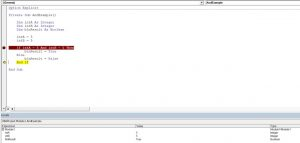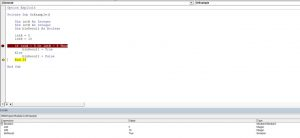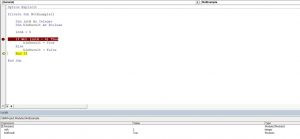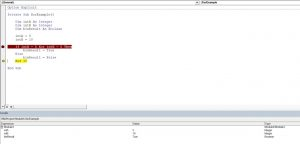# VBA Logical Operators – OR, AND, XOR, NOT, IS, & LIKE

VBA allows you to use the logical operators And, Or, Not, Xor to compare values. The operators are considered “Boolean”, which means they return True or False as a result.

If you want to learn how to compare strings, click here: VBA Compare Strings – StrComp

If you want to learn how to use comparison operators, click here: VBA Comparison Operators – Not Equal to & More

## Using the And Logical Operator

The And logical operator compares two or more conditions. If all the conditions are true, the operator will return True. If at least one of the conditions is not true, the operator will return False. Here is an example:

``````Dim intA As Integer
Dim intB As Integer
Dim blnResult As Boolean

intA = 5
intB = 5

If intA = 5 And intB = 5 Then
blnResult = True
Else
blnResult = False
End If``````

In this example, we want to check if both intA and intB are equal to 5. If this is true, the value of Boolean blnResult will be True, otherwise, it will be False.

First, we set values of intA and intB to 5:

``````intA = 5
intB = 5``````

After that, we use the And operator in the If statement to check if the values are equal to 5:

``````If intA = 5 And intB = 5 Then
blnResult = True
Else
blnResult = False
End If``````

As both variables are equal to 5, the blnResult returns True:Image 1. Using the And logical operator in VBA

## Using the Or Logical Operator

The Or logical operator compares two or more conditions. If at least one of the conditions is true, it will return True. If none of the conditions are true, the operator will return False. Here is the code for the example:

``````Dim intA As Integer
Dim intB As Integer
Dim blnResult As Boolean

intA = 5
intB = 10

If intA = 5 Or intB = 5 Then
blnResult = True
Else
blnResult = False
End If``````

In this example, we want to check if both intA is equal to 5. or intB is equal to 10. If any of these conditions is true, the value of Boolean blnResult will be True, otherwise, it will be False.

First, we set the value of intA to 5 and intB to 10:

``````intA = 5
intB = 10``````

After that, we use the Or operator in the If statement to check if any of the values is equal to 5:

``````If intA = 5 Or intB = 5 Then
blnResult = True
Else
blnResult = False
End If``````

As intA value is 5, the blnResult returns True:Image 2. Using the Or logical operator in VBA

## Using the Not Logical Operator

The Not logical operator checks one or more conditions. If the conditions are true, the operator returns False. Otherwise, it returns True. Here is the code for the example:

``````Dim intA As Integer
Dim blnResult As Boolean

intA = 5

If Not (intA = 6) Then
blnResult = True
Else
blnResult = False
End If``````

In this example, we want to check if the value of intA is not equal to 6. If intA is different than 6, the value of Boolean blnResult will be True, otherwise, it will be False.

First, we set the value of intA to 5:

``intA = 5``

After that, we use the Not operator in the If statement to check if the value of intA is different than 6:

``````If Not (intA = 6) Then
blnResult = True
Else
blnResult = False
End If``````

As intA value is 5, the blnResult returns True:Image 3. Using the Not logical operator in VBA

## Using the Xor Logical Operator

The Xor logical operator compares two or more conditions. If exactly one of the conditions is true, it will return True. If none of the conditions are true, or more than one are true, it will return False. Here is the code for the example:

``````Dim intA As Integer
Dim intB As Integer
Dim blnResult As Boolean

intA = 5
intB = 10

If intA = 5 Xor intB = 5 Then
blnResult = True
Else
blnResult = False
End If``````

In this example, we want to check if exactly one of the values (intA or IntB) are equal to 5. If only one condition is true, the value of Boolean blnResult will be True, otherwise, it will be False.

First, we set the value of intA to 5 and intB to 10:

``````intA = 5
intB = 10``````

After that, we use the Or operator in the If statement to check if any of the values is equal to 5:

``````If intA = 5 Xor intB = 5 Then
blnResult = True
Else
blnResult = False
End If``````

As intA value is 5 and intB is 10, the blnResult returns True:Image 4. Using the Xor logical operator in VBA

## Is Operator

The Is Operator tests if two object variables store the same object.

Let’s look at an example. Here we will assign two worksheets to worksheet objects rng1 and rng2, testing if the two worksheet objects store the same worksheet:

``````Sub CompareObjects()
Dim ws1 As Worksheet, ws2 As Worksheet

Set ws1 = Sheets("Sheet1")
Set ws2 = Sheets("Sheet2")

If ws1 Is ws2 Then
MsgBox "Same WS"
Else
MsgBox "Different WSs"
End If

End Sub``````

Of course the worksheet objects are not the same, so “Different WSs” is returned.

## Like Operator

The Like Operator can compare two strings for inexact matches. This example will test if a string starts with “Mr.”

``````Sub LikeDemo()

Dim strName As String
Dim blnResult As Boolean

strName = "Mr. Michael James"

If strName Like "Mr*" Then
blnResult = True
Else
blnResult = False
End If

End Sub``````

Stop searching for VBA code online. Learn more about AutoMacro - A VBA Code Builder that allows beginners to code procedures from scratch with minimal coding knowledge and with many time-saving features for all users!